Home > A2C > Chapter 9 > Lesson 9.3.2 > Problem9-156

9-156.
1. Rewrite each of the following division problems as a single complex number in simplest form. Homework Help ✎

1.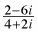2.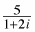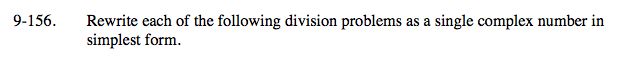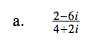First simplify the equation.

$\frac{1-3\textit{i}}{2+\textit{i}}$

Now multiply by the conjugate.

$\frac{(1-3\textit{i})(2-\textit{i})}{(2-\textit{i})(2+\textit{i})}$

$-\frac{1}{5}+\frac{-7}{5}i$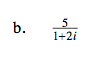See part (a).